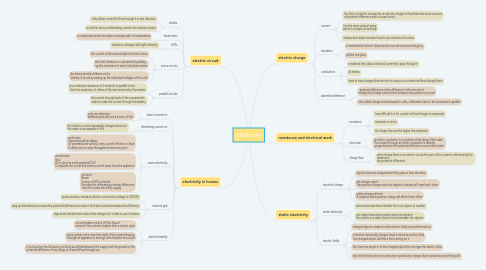# Electricity

Get Started. It's FreeElectricity## 1. electric charge

### 1.1. current

1.1.1. -the flow of electric charges for an electric charge to flow there has to be a source of potential difference and a closed circuit

1.1.2. has the same value at every point in a single closed loop

1.1.3. measured in amps ammeter have to be connected in series

### 1.2. insulators

1.2.1. A material that doesn't allow electric currents to pass through iy

1.2.2. plastic and glass

### 1.3. conductors

1.3.1. a material that allows electrical currents to pass through it

1.3.2. all metals

1.3.3. have to have charges that are free to move so a current can flow through them

### 1.4. potential difference

1.4.1. -potential difference is the difference in the amount of energy that charge carriers have between two points in a circuit

1.4.2. also called voltage and measured in volts, voltmeters have to be connected in parallel

## 2. resistance and electrical work

### 2.1. resistance

2.1.1. how difficult it is for current to flow through a component

2.1.2. measures in ohms

2.1.3. the longer the wire the higher the resistance

### 2.2. ohms law

2.2.1. an ohmic conductor is a conductor that obeys Ohm's law~ The current through an ohmic conductor is directly proportional to the potential difference across the resistor

### 2.3. charge flow

2.3.1. when charge flows in an electric circuit the size of the current is affected by the~ resistance the potential difference

## 3. electric circuit

### 3.1. diodes

3.1.1. -only allows current to flow through it in one direction

3.1.2. a rectifier turns an alternating current into a direct current

### 3.2. thermistors

3.2.1. a component where resistance changes with its temperature

### 3.3. LDRs

3.3.1. resistance changes with light intensity

### 3.4. series circuits

3.4.1. the current is the same at all point in the circuit

3.4.2. the total resistance is calculated by adding up the resistance of each individual resistor

3.4.3. the total potential difference of a battery is found by adding up the individual voltages of the cells

### 3.5. parallel circuits

3.5.1. the combined resistance of 2 resistors in parallel is less than the resistance of either of the two resistors by themselves

3.5.2. the current through each of the components adds to make the current through the battery

## 4. electricity in homes

### 4.1. direct current d.c

4.1.1. only one direction~ batteries and cells are a source of this

### 4.2. alternating current a.c

4.2.1. the electric current repeatedly changes direction~ the mains is an example of this

### 4.3. mains electricity

4.3.1. earth wire- Green and yellow stripes 0V potential and will only carry current if there is a fault A safety wire to stop the appliance becoming live

4.3.2. neutral wire- Blue At or close to earth potential (0V) Completes the circuit and carries current away from the appliance

4.3.3. live wire- Brown Carries a 230V potential Provides the alternating potential difference from the mains electricity supply

### 4.4. national grid

4.4.1. power stations create an electric current at a voltage of 250000

4.4.2. step up transformers increase the potential difference to reduce the heat lost and increases the efficiency

4.4.3. step down transformers reduce the voltage so it is able to use in homes

### 4.5. electrical safety

4.5.1. circuit breakers switch off the flow of current if the current is higher than a certain value

4.5.2. fuses contain a thin wire that melts if the current flowing through an appliance is too high which breaks the circuit

4.5.3. if you touches the live wire your body would link between the supply and the ground so the potential difference if very large so it would flow through you

## 5. static electricity

### 5.1. electrical charge

5.1.1. objects become charged when they gain or lose electrons

5.1.2. like charges repel- Two positive charges and two negative charges will repel each other

5.1.3. unlike charges attract- A negative and a positive charge will attract each other

### 5.2. static electricity

5.2.1. arises when electrons transfer from one object to another

5.2.2. one object becomes positive and one negative- this results in a static electric force between the objects

### 5.3. electric fields

5.3.1. charged objects create invisible electric fields around themselves

5.3.2. if another electrically charged object enters an electric field the charged object will feel a force acting on it

5.3.3. the closer an object is to the charged object the stronger the electric field

5.3.4. electric field lines show the direction a positively charges object would move at that point